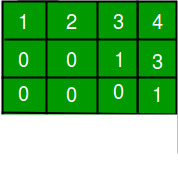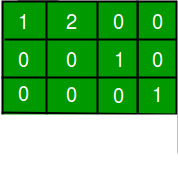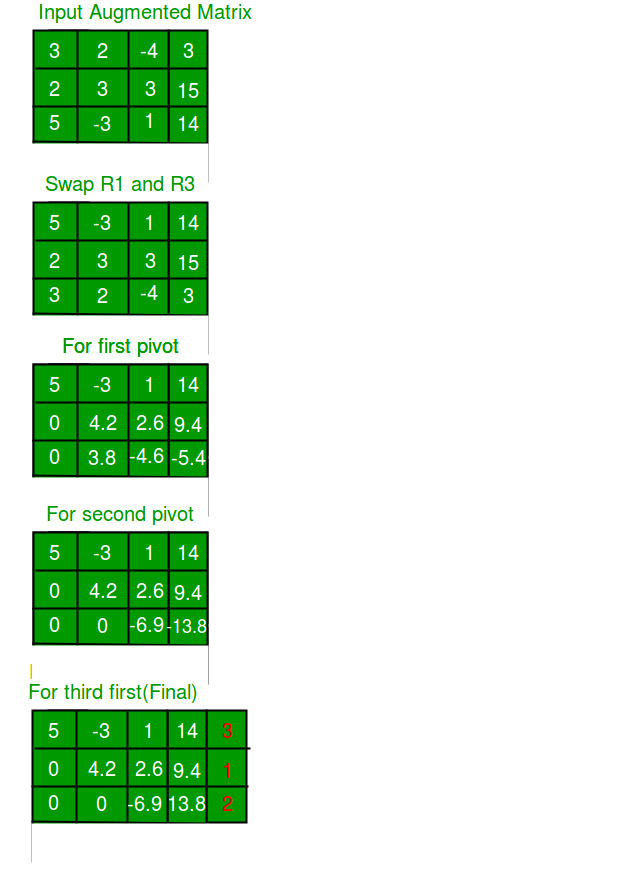Open in App
Not now

# Gaussian Elimination to Solve Linear Equations

• Difficulty Level : Medium
• Last Updated : 08 Nov, 2022

The article focuses on using an algorithm for solving a system of linear equations. We will deal with the matrix of coefficients. Gaussian Elimination does not work on singular matrices (they lead to division by zero).

```Input: For N unknowns, input is an augmented
matrix of size N x (N+1). One extra
column is for Right Hand Side (RHS)
mat[N][N+1] = {{3.0, 2.0,-4.0, 3.0},
{2.0, 3.0, 3.0, 15.0},
{5.0, -3, 1.0, 14.0}
};
Output: Solution to equations is:
3.000000
1.000000
2.000000

Explanation:
Given matrix represents following equations
3.0X1 + 2.0X2 - 4.0X3 =  3.0
2.0X1 + 3.0X2 + 3.0X3 = 15.0
5.0X1 - 3.0X2 +    X3 = 14.0

There is a unique solution for given equations,
solutions is, X1 = 3.0, X2 = 1.0, X3 = 2.0, ```Row echelon form: Matrix is said to be in r.e.f. if the following conditions hold:

1. The first non-zero element in each row, called the leading coefficient, is 1.
2. Each leading coefficient is in a column to the right of the previous row leading coefficient.
3. Rows with all zeros are below rows with at least one non-zero element.Reduced row echelon form: Matrix is said to be in r.r.e.f. if the following conditions hold –

1. All the conditions for r.e.f.
2. The leading coefficient in each row is the only non-zero entry in its column.

The algorithm is majorly about performing a sequence of operations on the rows of the matrix. What we would like to keep in mind while performing these operations is that we want to convert the matrix into an upper triangular matrix in row echelon form. The operations can be:

1. Swapping two rows
2. Multiplying a row by a non-zero scalar
3. Adding to one row a multiple of another

The process:

1. Forward elimination: reduction to row echelon form. Using it one can tell whether there are no solutions, or unique solution, or infinitely many solutions.
2. Back substitution: further reduction to reduced row echelon form.

Algorithm:

1. Partial pivoting: Find the kth pivot by swapping rows, to move the entry with the largest absolute value to the pivot position. This imparts computational stability to the algorithm.
2. For each row below the pivot, calculate the factor f which makes the kth entry zero, and for every element in the row subtract the fth multiple of the corresponding element in the kth row.
3. Repeat above steps for each unknown. We will be left with a partial r.e.f. matrix.

Below is the implementation of the above algorithm.

## C++

 `// C++ program to demonstrate working of Gaussian Elimination``// method``#include``using` `namespace` `std;` `#define N 3        // Number of unknowns` `// function to reduce matrix to r.e.f.  Returns a value to``// indicate whether matrix is singular or not``int` `forwardElim(``double` `mat[N][N+1]);` `// function to calculate the values of the unknowns``void` `backSub(``double` `mat[N][N+1]);` `// function to get matrix content``void` `gaussianElimination(``double` `mat[N][N+1])``{``    ``/* reduction into r.e.f. */``    ``int` `singular_flag = forwardElim(mat);` `    ``/* if matrix is singular */``    ``if` `(singular_flag != -1)``    ``{``        ``printf``(``"Singular Matrix.\n"``);` `        ``/* if the RHS of equation corresponding to``           ``zero row  is 0, * system has infinitely``           ``many solutions, else inconsistent*/``        ``if` `(mat[singular_flag][N])``            ``printf``(``"Inconsistent System."``);``        ``else``            ``printf``(``"May have infinitely many "``                   ``"solutions."``);` `        ``return``;``    ``}` `    ``/* get solution to system and print it using``       ``backward substitution */``    ``backSub(mat);``}` `// function for elementary operation of swapping two rows``void` `swap_row(``double` `mat[N][N+1], ``int` `i, ``int` `j)``{``    ``//printf("Swapped rows %d and %d\n", i, j);` `    ``for` `(``int` `k=0; k<=N; k++)``    ``{``        ``double` `temp = mat[i][k];``        ``mat[i][k] = mat[j][k];``        ``mat[j][k] = temp;``    ``}``}` `// function to print matrix content at any stage``void` `print(``double` `mat[N][N+1])``{``    ``for` `(``int` `i=0; i v_max)``                ``v_max = mat[i][k], i_max = i;` `        ``/* if a principal diagonal element  is zero,``         ``* it denotes that matrix is singular, and``         ``* will lead to a division-by-zero later. */``        ``if` `(!mat[k][i_max])``            ``return` `k; ``// Matrix is singular` `        ``/* Swap the greatest value row with current row */``        ``if` `(i_max != k)``            ``swap_row(mat, k, i_max);`  `        ``for` `(``int` `i=k+1; i= 0; i--)``    ``{``        ``/* start with the RHS of the equation */``        ``x[i] = mat[i][N];` `        ``/* Initialize j to i+1 since matrix is upper``           ``triangular*/``        ``for` `(``int` `j=i+1; j

## Java

 `// Java program to demonstrate working of Gaussian Elimination``// method``import` `java.io.*;``class` `GFG``{` `  ``public` `static` `int` `N = ``3``; ``// Number of unknowns` `  ``// function to get matrix content``  ``static` `void` `gaussianElimination(``double` `mat[][])``  ``{` `    ``/* reduction into r.e.f. */``    ``int` `singular_flag = forwardElim(mat);` `    ``/* if matrix is singular */``    ``if` `(singular_flag != -``1``)``    ``{``      ``System.out.println(``"Singular Matrix."``);` `      ``/* if the RHS of equation corresponding to``               ``zero row  is 0, * system has infinitely``               ``many solutions, else inconsistent*/``      ``if` `(mat[singular_flag][N] != ``0``)``        ``System.out.print(``"Inconsistent System."``);``      ``else``        ``System.out.print(``        ``"May have infinitely many solutions."``);` `      ``return``;``    ``}` `    ``/* get solution to system and print it using``           ``backward substitution */``    ``backSub(mat);``  ``}` `  ``// function for elementary operation of swapping two``  ``// rows``  ``static` `void` `swap_row(``double` `mat[][], ``int` `i, ``int` `j)``  ``{``    ``// printf("Swapped rows %d and %d\n", i, j);` `    ``for` `(``int` `k = ``0``; k <= N; k++)``    ``{``      ``double` `temp = mat[i][k];``      ``mat[i][k] = mat[j][k];``      ``mat[j][k] = temp;``    ``}``  ``}` `  ``// function to print matrix content at any stage``  ``static` `void` `print(``double` `mat[][])``  ``{``    ``for` `(``int` `i = ``0``; i < N; i++, System.out.println())``      ``for` `(``int` `j = ``0``; j <= N; j++)``        ``System.out.print(mat[i][j]);``    ``System.out.println();``  ``}` `  ``// function to reduce matrix to r.e.f.``  ``static` `int` `forwardElim(``double` `mat[][])``  ``{``    ``for` `(``int` `k = ``0``; k < N; k++)``    ``{` `      ``// Initialize maximum value and index for pivot``      ``int` `i_max = k;``      ``int` `v_max = (``int``)mat[i_max][k];` `      ``/* find greater amplitude for pivot if any */``      ``for` `(``int` `i = k + ``1``; i < N; i++)``        ``if` `(Math.abs(mat[i][k]) > v_max)``        ``{``          ``v_max = (``int``)mat[i][k];``          ``i_max = i;``        ``}` `      ``/* if a principal diagonal element  is zero,``             ``* it denotes that matrix is singular, and``             ``* will lead to a division-by-zero later. */``      ``if` `(mat[k][i_max] == ``0``)``        ``return` `k; ``// Matrix is singular` `      ``/* Swap the greatest value row with current row``             ``*/``      ``if` `(i_max != k)``        ``swap_row(mat, k, i_max);` `      ``for` `(``int` `i = k + ``1``; i < N; i++)``      ``{` `        ``/* factor f to set current row kth element``                 ``* to 0, and subsequently remaining kth``                 ``* column to 0 */``        ``double` `f = mat[i][k] / mat[k][k];` `        ``/* subtract fth multiple of corresponding``                   ``kth row element*/``        ``for` `(``int` `j = k + ``1``; j <= N; j++)``          ``mat[i][j] -= mat[k][j] * f;` `        ``/* filling lower triangular matrix with``                 ``* zeros*/``        ``mat[i][k] = ``0``;``      ``}` `      ``// print(mat);        //for matrix state``    ``}` `    ``// print(mat);            //for matrix state``    ``return` `-``1``;``  ``}` `  ``// function to calculate the values of the unknowns``  ``static` `void` `backSub(``double` `mat[][])``  ``{``    ``double` `x[]``      ``= ``new` `double``[N]; ``// An array to store solution` `    ``/* Start calculating from last equation up to the``           ``first */``    ``for` `(``int` `i = N - ``1``; i >= ``0``; i--)``    ``{` `      ``/* start with the RHS of the equation */``      ``x[i] = mat[i][N];` `      ``/* Initialize j to i+1 since matrix is upper``               ``triangular*/``      ``for` `(``int` `j = i + ``1``; j < N; j++)``      ``{` `        ``/* subtract all the lhs values``                 ``* except the coefficient of the variable``                 ``* whose value is being calculated */``        ``x[i] -= mat[i][j] * x[j];``      ``}` `      ``/* divide the RHS by the coefficient of the``               ``unknown being calculated */``      ``x[i] = x[i] / mat[i][i];``    ``}` `    ``System.out.println();``    ``System.out.println(``"Solution for the system:"``);``    ``for` `(``int` `i = ``0``; i < N; i++)``    ``{``      ``System.out.format(``"%.6f"``, x[i]);``      ``System.out.println();``    ``}``  ``}` `  ``// Driver program``  ``public` `static` `void` `main(String[] args)``  ``{` `    ``/* input matrix */``    ``double` `mat[][] = { { ``3.0``, ``2.0``, -``4.0``, ``3.0` `},``                      ``{ ``2.0``, ``3.0``, ``3.0``, ``15.0` `},``                      ``{ ``5.0``, -``3``, ``1.0``, ``14.0` `} };` `    ``gaussianElimination(mat);``  ``}``}` `// This code is contributed by Dharanendra L V.`

## Python3

 `# Python3 program to demonstrate working of``# Gaussian Elimination method``N ``=` `3` `# function to get matrix content``def` `gaussianElimination(mat):` `    ``# reduction into r.e.f.``    ``singular_flag ``=` `forwardElim(mat)` `    ``# if matrix is singular``    ``if` `(singular_flag !``=` `-``1``):` `        ``print``(``"Singular Matrix."``)` `        ``# if the RHS of equation corresponding to``        ``#   zero row  is 0, * system has infinitely``        ``#   many solutions, else inconsistent*/``        ``if` `(mat[singular_flag][N]):``            ``print``(``"Inconsistent System."``)``        ``else``:``            ``print``(``"May have infinitely many solutions."``)` `        ``return` `    ``# get solution to system and print it using``    ``#   backward substitution``    ``backSub(mat)` `# function for elementary operation of swapping two rows``def` `swap_row(mat, i, j):` `    ``for` `k ``in` `range``(N ``+` `1``):` `        ``temp ``=` `mat[i][k]``        ``mat[i][k] ``=` `mat[j][k]``        ``mat[j][k] ``=` `temp` `# function to reduce matrix to r.e.f.``def` `forwardElim(mat):``    ``for` `k ``in` `range``(N):``      ` `        ``# Initialize maximum value and index for pivot``        ``i_max ``=` `k``        ``v_max ``=` `mat[i_max][k]` `        ``# find greater amplitude for pivot if any``        ``for` `i ``in` `range``(k ``+` `1``, N):``            ``if` `(``abs``(mat[i][k]) > v_max):``                ``v_max ``=` `mat[i][k]``                ``i_max ``=` `i` `        ``# if a principal diagonal element  is zero,``        ``# it denotes that matrix is singular, and``        ``# will lead to a division-by-zero later.``        ``if` `not` `mat[k][i_max]:``            ``return` `k    ``# Matrix is singular` `        ``# Swap the greatest value row with current row``        ``if` `(i_max !``=` `k):``            ``swap_row(mat, k, i_max)` `        ``for` `i ``in` `range``(k ``+` `1``, N):` `            ``# factor f to set current row kth element to 0,``            ``# and subsequently remaining kth column to 0 */``            ``f ``=` `mat[i][k]``/``mat[k][k]` `            ``# subtract fth multiple of corresponding kth``            ``# row element*/``            ``for` `j ``in` `range``(k ``+` `1``, N ``+` `1``):``                ``mat[i][j] ``-``=` `mat[k][j]``*``f` `            ``# filling lower triangular matrix with zeros*/``            ``mat[i][k] ``=` `0` `        ``# print(mat);        //for matrix state` `    ``# print(mat);            //for matrix state``    ``return` `-``1` `# function to calculate the values of the unknowns``def` `backSub(mat):` `    ``x ``=` `[``None` `for` `_ ``in` `range``(N)]    ``# An array to store solution` `    ``# Start calculating from last equation up to the``    ``#  first */``    ``for` `i ``in` `range``(N``-``1``, ``-``1``, ``-``1``):` `        ``# start with the RHS of the equation */``        ``x[i] ``=` `mat[i][N]` `        ``# Initialize j to i+1 since matrix is upper``        ``#  triangular*/``        ``for` `j ``in` `range``(i ``+` `1``, N):``          ` `            ``# subtract all the lhs values``            ``# except the coefficient of the variable``            ``# whose value is being calculated */``            ``x[i] ``-``=` `mat[i][j]``*``x[j]` `        ``# divide the RHS by the coefficient of the``        ``#  unknown being calculated``        ``x[i] ``=` `(x[i]``/``mat[i][i])` `    ``print``(``"\nSolution for the system:"``)``    ``for` `i ``in` `range``(N):``        ``print``(``"{:.8f}"``.``format``(x[i]))` `# Driver program` `# input matrix``mat ``=` `[[``3.0``, ``2.0``, ``-``4.0``, ``3.0``], [``2.0``, ``3.0``, ``3.0``, ``15.0``], [``5.0``, ``-``3``, ``1.0``, ``14.0``]]``gaussianElimination(mat)` `# This code is contributed by phasing17`

## C#

 `// C# program to demonstrate working``// of Gaussian Elimination method``using` `System;` `class` `GFG{``    ` `// Number of unknowns``public` `static` `int` `N = 3;` `// Function to get matrix content``static` `void` `gaussianElimination(``double` `[,]mat)``{` `    ``/* reduction into r.e.f. */``    ``int` `singular_flag = forwardElim(mat);``    ` `    ``/* if matrix is singular */``    ``if` `(singular_flag != -1)``    ``{``        ``Console.WriteLine(``"Singular Matrix."``);``        ` `        ``/* if the RHS of equation corresponding to``           ``zero row  is 0, * system has infinitely``           ``many solutions, else inconsistent*/``        ``if` `(mat[singular_flag,N] != 0)``            ``Console.Write(``"Inconsistent System."``);``        ``else``            ``Console.Write(``"May have infinitely "` `+``                          ``"many solutions."``);``        ` `        ``return``;``    ``}``    ` `    ``/* get solution to system and print it using``    ``backward substitution */``    ``backSub(mat);``}` `// Function for elementary operation of swapping two``// rows``static` `void` `swap_row(``double` `[,]mat, ``int` `i, ``int` `j)``{``    ` `    ``// printf("Swapped rows %d and %d\n", i, j);``    ` `    ``for``(``int` `k = 0; k <= N; k++)``    ``{``        ``double` `temp = mat[i, k];``        ``mat[i, k] = mat[j, k];``        ``mat[j, k] = temp;``    ``}``}``    ` `// Function to print matrix content at any stage``static` `void` `print(``double` `[,]mat)``{``    ``for``(``int` `i = 0; i < N; i++, Console.WriteLine())``        ``for``(``int` `j = 0; j <= N; j++)``            ``Console.Write(mat[i, j]);``            ` `    ``Console.WriteLine();``}` `// Function to reduce matrix to r.e.f.``static` `int` `forwardElim(``double` `[,]mat)``{``    ``for``(``int` `k = 0; k < N; k++)``    ``{``        ` `        ``// Initialize maximum value and index for pivot``        ``int` `i_max = k;``        ``int` `v_max = (``int``)mat[i_max, k];``        ` `        ``/* find greater amplitude for pivot if any */``        ``for``(``int` `i = k + 1; i < N; i++)``        ``{``            ``if` `(Math.Abs(mat[i, k]) > v_max)``            ``{``                ``v_max = (``int``)mat[i, k];``                ``i_max = i;``            ``}``        ` `            ``/* If a principal diagonal element  is zero,``            ``*  it denotes that matrix is singular, and``            ``*  will lead to a division-by-zero later. */``            ``if` `(mat[k, i_max] == 0)``                ``return` `k; ``// Matrix is singular``            ` `            ``/* Swap the greatest value row with``               ``current row``            ``*/``            ``if` `(i_max != k)``                ``swap_row(mat, k, i_max);``            ` `            ``for``(``int` `a = k + 1; a < N; a++)``            ``{``            ` `                ``/* factor f to set current row kth element``                ``*  to 0, and subsequently remaining kth``                ``*  column to 0 */``                ``double` `f = mat[i, k] / mat[k, k];``                ` `                ``/* subtract fth multiple of corresponding``                   ``kth row element*/``                ``for``(``int` `j = k + 1; j <= N; j++)``                    ``mat[i, j] -= mat[k, j] * f;``                ` `                ``/* filling lower triangular matrix with``                ``*  zeros*/``                ``mat[i, k] = 0;``            ``}``        ``}``        ``// print(mat);        //for matrix state``    ``}``    ` `    ``// print(mat);            //for matrix state``    ``return` `-1;``}` `// Function to calculate the values of the unknowns``static` `void` `backSub(``double` `[,]mat)``{``    ` `    ``// An array to store solution``    ``double` `[]x = ``new` `double``[N];``    ` `    ``/* Start calculating from last equation up to the``       ``first */``    ``for``(``int` `i = N - 1; i >= 0; i--)``    ``{``    ` `        ``/* start with the RHS of the equation */``        ``x[i] = mat[i,N];``        ` `        ``/* Initialize j to i+1 since matrix is upper``        ``triangular*/``        ``for``(``int` `j = i + 1; j < N; j++)``        ``{``        ` `            ``/* subtract all the lhs values``             ``* except the coefficient of the variable``             ``* whose value is being calculated */``            ``x[i] -= mat[i,j] * x[j];``        ``}``        ` `        ``/* divide the RHS by the coefficient of the``        ``unknown being calculated */``        ``x[i] = x[i] / mat[i,i];``    ``}``    ` `    ``Console.WriteLine();``    ``Console.WriteLine(``"Solution for the system:"``);``    ``for``(``int` `i = 0; i < N; i++)``    ``{``        ``Console.Write(``"{0:F6}"``, x[i]);``        ``Console.WriteLine();``    ``}``}` `// Driver code``public` `static` `void` `Main(String[] args)``{``    ` `    ``/* input matrix */``    ``double` `[,]mat = { { 3.0, 2.0, -4.0, 3.0 },``                      ``{ 2.0, 3.0, 3.0, 15.0 },``                      ``{ 5.0, -3, 1.0, 14.0 } };``    ` `    ``gaussianElimination(mat);``}``}` `// This code is contributed by shikhasingrajput`

## PHP

 ` ``\$v_max``)``                ``{``                    ``\$v_max` `= ``\$mat``[``\$i``][``\$k``];``                    ``\$i_max` `= ``\$i``;``                ``}` `        ``/* if a principal diagonal element is zero,``        ``* it denotes that matrix is singular, and``        ``* will lead to a division-by-zero later. */``        ``if` `(!``\$mat``[``\$k``][``\$i_max``])``            ``return` `\$k``; ``// Matrix is singular` `        ``/* Swap the greatest value row with current row */``        ``if` `(``\$i_max` `!= ``\$k``)``            ``swap_row(``\$mat``, ``\$k``, ``\$i_max``);`  `        ``for` `(``\$i` `= ``\$k` `+ 1; ``\$i` `< ``\$N``; ``\$i``++)``        ``{``            ``/* factor f to set current row kth element to 0,``            ``* and subsequently remaining kth column to 0 */``            ``\$f` `= ``\$mat``[``\$i``][``\$k``]/``\$mat``[``\$k``][``\$k``];` `            ``/* subtract fth multiple of corresponding kth``            ``row element*/``            ``for` `(``\$j` `= ``\$k` `+ 1; ``\$j` `<= ``\$N``; ``\$j``++)``                ``\$mat``[``\$i``][``\$j``] -= ``\$mat``[``\$k``][``\$j``] * ``\$f``;` `            ``/* filling lower triangular matrix with zeros*/``            ``\$mat``[``\$i``][``\$k``] = 0;``        ``}` `        ``//print(mat); //for matrix state``    ``}``    ``//print(mat);     //for matrix state``    ``return` `-1;``}` `// function to calculate the values of the unknowns``function` `backSub(&``\$mat``)``{``    ``global` `\$N``;``    ``\$x` `= ``array_fill``(0, ``\$N``, 0); ``// An array to store solution` `    ``/* Start calculating from last equation up to the``    ``first */``    ``for` `(``\$i` `= ``\$N` `- 1; ``\$i` `>= 0; ``\$i``--)``    ``{``        ``/* start with the RHS of the equation */``        ``\$x``[``\$i``] = ``\$mat``[``\$i``][``\$N``];` `        ``/* Initialize j to i+1 since matrix is upper``        ``triangular*/``        ``for` `(``\$j` `= ``\$i` `+ 1; ``\$j` `< ``\$N``; ``\$j``++)``        ``{``            ``/* subtract all the lhs values``            ``* except the coefficient of the variable``            ``* whose value is being calculated */``            ``\$x``[``\$i``] -= ``\$mat``[``\$i``][``\$j``] * ``\$x``[``\$j``];``        ``}` `        ``/* divide the RHS by the coefficient of the``        ``unknown being calculated */``        ``\$x``[``\$i``] = ``\$x``[``\$i``] / ``\$mat``[``\$i``][``\$i``];``    ``}` `    ``print``(``"\nSolution for the system:\n"``);``    ``for` `(``\$i` `= 0; ``\$i` `< ``\$N``; ``\$i``++)``        ``print``(number_format(``strval``(``\$x``[``\$i``]), 6).``"\n"``);``}` `// Driver program` `    ``/* input matrix */``    ``\$mat` `= ``array``(``array``(3.0, 2.0,-4.0, 3.0),``                        ``array``(2.0, 3.0, 3.0, 15.0),``                        ``array``(5.0, -3, 1.0, 14.0));` `    ``gaussianElimination(``\$mat``);` `// This code is contributed by mits``?>`

## Javascript

 `// JavaScript program to demonstrate working of Gaussian Elimination``// method` `let N = 3;` `// function to get matrix content``function` `gaussianElimination(mat)``{``    ``/* reduction into r.e.f. */``    ``let singular_flag = forwardElim(mat);` `    ``/* if matrix is singular */``    ``if` `(singular_flag != -1)``    ``{``        ``console.log(``"Singular Matrix."``);` `        ``/* if the RHS of equation corresponding to``           ``zero row  is 0, * system has infinitely``           ``many solutions, else inconsistent*/``        ``if` `(mat[singular_flag][N])``            ``console.log(``"Inconsistent System."``);``        ``else``            ``console.log(``"May have infinitely many solutions."``);` `        ``return``;``    ``}` `    ``/* get solution to system and print it using``       ``backward substitution */``    ``backSub(mat);``}` `// function for elementary operation of swapping two rows``function` `swap_row(mat, i, j)``{``    ``//printf("Swapped rows %d and %d\n", i, j);` `    ``for` `(``var` `k=0; k<=N; k++)``    ``{``        ``let temp = mat[i][k];``        ``mat[i][k] = mat[j][k];``        ``mat[j][k] = temp;``    ``}``}` `// function to print matrix content at any stage``function` `print(mat)``{``    ``for` `(``var` `i=0; i v_max)``                ``v_max = mat[i][k], i_max = i;` `        ``/* if a principal diagonal element  is zero,``         ``* it denotes that matrix is singular, and``         ``* will lead to a division-by-zero later. */``        ``if` `(!mat[k][i_max])``            ``return` `k; ``// Matrix is singular` `        ``/* Swap the greatest value row with current row */``        ``if` `(i_max != k)``            ``swap_row(mat, k, i_max);`  `        ``for` `(``var` `i=k+1; i= 0; i--)``    ``{``        ``/* start with the RHS of the equation */``        ``x[i] = mat[i][N];` `        ``/* Initialize j to i+1 since matrix is upper``           ``triangular*/``        ``for` `(``var` `j=i+1; j

Output

```Solution for the system:
3.000000
1.000000
2.000000
```

Illustration:Time Complexity: Since for each pivot we traverse the part to its right for each row below it, O(n)*(O(n)*O(n)) = O(n3).
We can also apply Gaussian Elimination for calculating:

1. Rank of a matrix
2. Determinant of a matrix
3. Inverse of an invertible square matrix